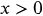1
54
views
54a
Problem

Problem 54a

Chapter 1: Functions and ModelsTextbook ExpertVerified Tutor
18 Oct 2021

Given information

The inequality is provided below.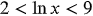Step-by-step explanation

Step 1.

Apply the concept of relationship between natural logarithm and exponential function

The natural logarithm is obtained when logarithm function is used with base e.

Mathematically, it is expressed below.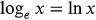The expression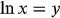is equivalent to the expression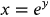Some important results are provided below.

1.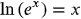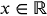2.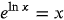,